x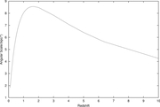Angular diameter distanceEncyclopedia
The angular diameter distance is a distance measure used in astronomy
Astronomy
Astronomy is a natural science that deals with the study of celestial objects and phenomena that originate outside the atmosphere of Earth...

. The angular diameter distance to an object is defined in terms of the object's actual size,, and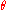the angular size of the object as viewed from earth.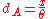The angular diameter distance depends on the assumed cosmology
Physical cosmology
Physical cosmology, as a branch of astronomy, is the study of the largest-scale structures and dynamics of the universe and is concerned with fundamental questions about its formation and evolution. For most of human history, it was a branch of metaphysics and religion...

of the universe. The angular diameter distance to an object at redshift
Redshift
In physics , redshift happens when light seen coming from an object is proportionally increased in wavelength, or shifted to the red end of the spectrum...

,, is expressed in terms of the comoving distance
Comoving distance
In standard cosmology, comoving distance and proper distance are two closely related distance measures used by cosmologists to define distances between objects...

,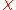as: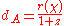Where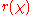is defined as: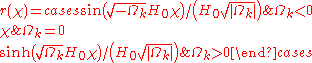Where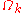is the curvature density and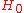is the value of the Hubble parameter today.

In the currently favoured geometric model of our Universe
Lambda-CDM model
ΛCDM or Lambda-CDM is an abbreviation for Lambda-Cold Dark Matter, which is also known as the cold dark matter model with dark energy...

, the "angular diameter distance" of an object is a good approximation to the "real distance", i.e. the proper distance
Comoving distance
In standard cosmology, comoving distance and proper distance are two closely related distance measures used by cosmologists to define distances between objects...

when the light left the object. Note that beyond a certain redshift
Redshift
In physics , redshift happens when light seen coming from an object is proportionally increased in wavelength, or shifted to the red end of the spectrum...

, the angular diameter distance gets smaller with increasing redshift
Redshift
In physics , redshift happens when light seen coming from an object is proportionally increased in wavelength, or shifted to the red end of the spectrum...

. In other words an object "behind" another of the same size, beyond a certain redshift (roughly z=1.5), appears larger on the sky, and would therefore have a smaller "angular diameter distance".

## Angular size redshift relation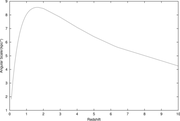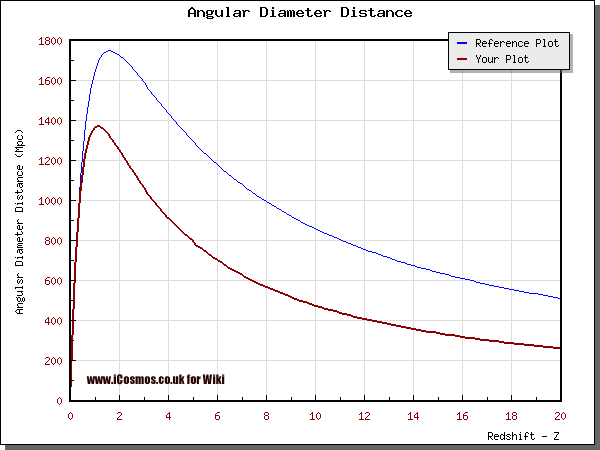The angular size redshift relation describes the relation between the angular size observed on the sky of an object of given physical size, and the objects redshift
Redshift
In physics , redshift happens when light seen coming from an object is proportionally increased in wavelength, or shifted to the red end of the spectrum...

from Earth
Earth
Earth is the third planet from the Sun, and the densest and fifth-largest of the eight planets in the Solar System. It is also the largest of the Solar System's four terrestrial planets...

(which is related to its distance,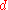, from Earth). In a Euclidean
Euclidean
Euclidean relates to Euclid , a town or others. It may refer to:Geometry...

geometry the relation between size on the sky and distance from Earth would simply be given by the equation: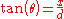whereis the angular size of the object on the sky,is the size of the object andis the distance to the object. Whereis small this approximates to: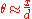.

However, in the currently favoured geometric model of our Universe
Lambda-CDM model
ΛCDM or Lambda-CDM is an abbreviation for Lambda-Cold Dark Matter, which is also known as the cold dark matter model with dark energy...

, the relation is more complicated. In this model, objects at redshift
Redshift
In physics , redshift happens when light seen coming from an object is proportionally increased in wavelength, or shifted to the red end of the spectrum...

s greater than about 1.5 appear larger on the sky with increasing redshift
Redshift
In physics , redshift happens when light seen coming from an object is proportionally increased in wavelength, or shifted to the red end of the spectrum...

.

This is related to the angular diameter distance, which is the distance an object is calculated to be at fromand, assuming the Universe is Euclidean
Euclidean
Euclidean relates to Euclid , a town or others. It may refer to:Geometry...

.

The actual relation between the angular-diameter distance,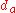, and redshift is given below.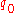is called the deceleration parameter and measures the deceleration of the expansion rate of the Universe; in the simplest models,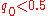corresponds to the case where the Universe will expand for ever,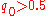to closed models which will ultimately stop expanding and contract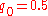corresponds to the critical case – Universes which will just be able to expand to infinity without re-contracting.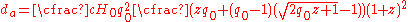The Mattig relation yields the angular-diameter distance as a function of redshift for a universe with ΩΛ = 0.
The source of this article is wikipedia, the free encyclopedia.  The text of this article is licensed under the GFDL.